Fingerings Home Octave 1: F  (C) Gb  (Db) G  (D) Ab  (Eb) A  (E) Bb  (F) B  (Gb) C  (G) Db  (Ab) D  (A) Eb  (Bb) E  (B) Octave 2+: F  (C) Gb  (Db) G  (D) Ab  (Eb) A  (E) Bb  (F) B  (Gb) C  (G) Db  (Ab) D  (A) Eb  (Bb) E  (B) F  (C) Gb  (Db) G  (D)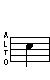C (G) - 1st Octave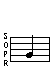## Trills

Trill - From D   Trill - From Db
D C   Db C (alt)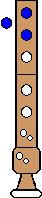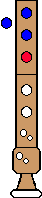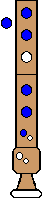Top

## Turned Trills

Turned Trill
Full step above, Full step below
D C Bb C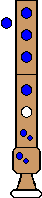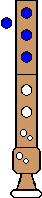Top

Turned Trill
Half step above; Full step below
Db C (alt) Bb CTop

Turned Trill
Full step above; Half step below
D C B C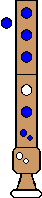Top

Turned Trill
Half step above; Half step below
Db C (alt) B CTop

## Regular Turns

Turn
Full step above; Full step below
D C Bb CTop

Turn
Half step above; Full step below
Db C Bb CComments: All normal fingerings - note the contrast between this and the turned trill
Top

Turn
Full step above; Half step below
D C B C